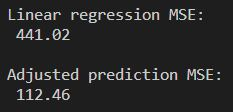Garrett Mayock's Blog

# Adjusting Linear Regression Models for Seasonality

### Following along Managing Supply Chain Operations Chapter 2 using Python, cont'd.Garrett Mayock posted 2019-03-06 02:07:52 UTC

As before: I am reading Managing Supply Chain Operations. Chapter 2 details Forecasting and Demand Management methods. I figured I'd kill two birds with one stone (learning the material and learning Python) by recreating the chapter material in Python. I anticipate answering the chapter exercises in Python as well, but will save that for another blog post. (Writer's note - please look at the other Chapter 2 blogs (naivenon-naivelinear regression, Holt's model, fixing inefficient optimization code). Click here to download the Excel file I ingested.

What does seasonal data look like?

Seasonal data is data which has periodic behavior.

It's probably easiest to think of in terms of weather. There's a joke in the Midwest that they have two seasons - winter and construction - and that's because activities like digging foundations or building roads is much more difficult when the ground is frozen. But because of that, it's easy to find seasonality in construction data. For illustrative purposes, let's look at the housing starts data over the past 20 years:

```import pandas as pd
import matplotlib.pyplot as plt

last_20_years_df = df[-80:]
plt.plot(last_20_years_df['observation_date'],last_20_years_df['HOUST1FQ'])
plt.xlabel('Year')
plt.ylabel('Housing Starts')
plt.title('Housing starts by quarter, last 20 years, ending Fall 2018')
plt.show()
```

Which produces: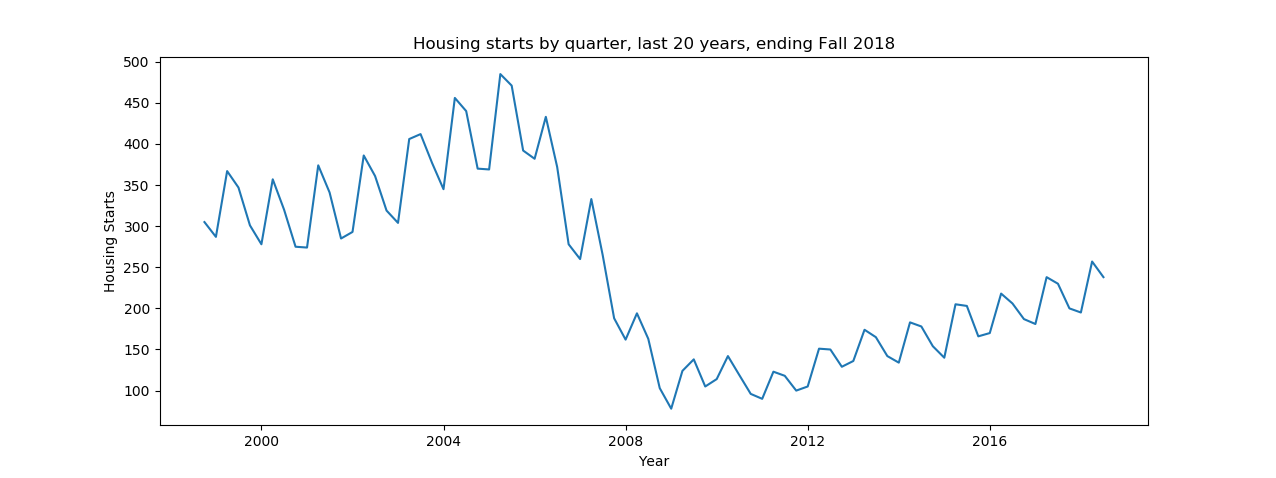Data source: https://fred.stlouisfed.org/series/houst1fq

To make it more clear, let's look at a bar chart which plots the percent of housing starts per quarter for each year. Matplotlib is a bit picky when it comes to bar charts. You have to declare width as a time delta when x is of type datetime - else it throws a TypeError because the default width (0.8) can not be added to a datetime value: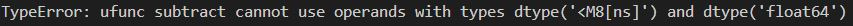Here's code that works and adds a specific color to each season for the last 20 full years:

```# This creates a totals column which shows the total for that year
df['year_total'] = df['HOUST1FQ'].groupby(df['observation_date'].dt.year).transform('sum')

# This creates a column which divides the housing starts for the given quarter by the total for that year
df['percent_of_year'] = df['HOUST1FQ']/df['year_total']

# These rows map a season value based on the month
season_map = {1:'Winter', 4:'Spring',7:'Summer',10:'Fall'}
df['season'] = df['observation_date'].dt.month.map(season_map)

﻿# These rows create a bar plot colored by season for the last 20 full years
last_20_full_years_df = df[-83:-3]
plt.legend(bbox_to_anchor=(0., 1.01, 1., .102), loc=3,ncol=4, mode="expand", borderaxespad=0.)
plt.xlabel('Year')
plt.ylabel('Housing Starts')
plt.title('Housing starts by quarter, last 20 full years (1997-2017)', y=1.07)
plt.show()
```

That results in: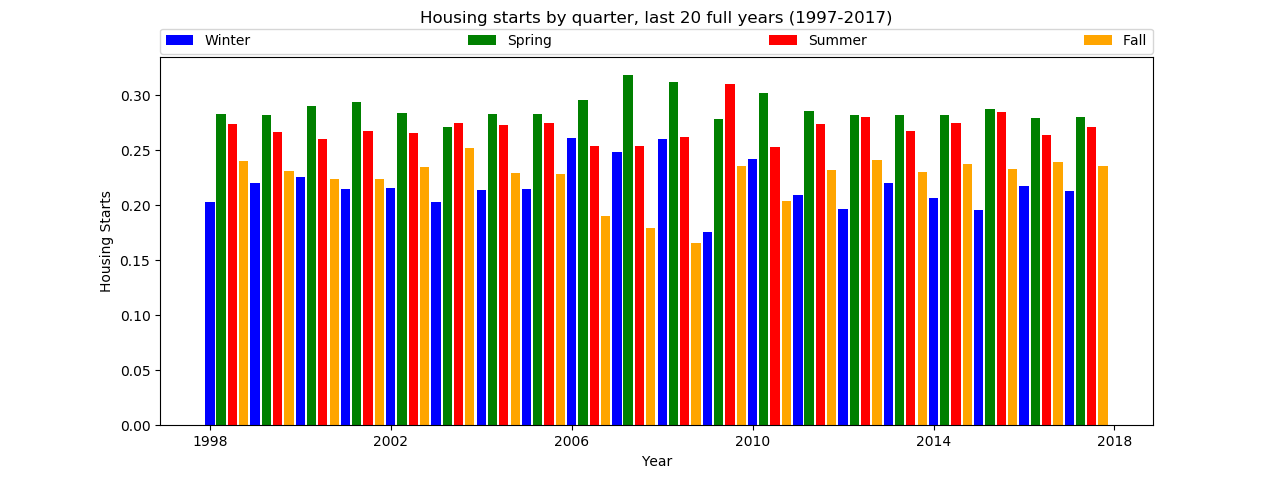Note - the legend is based on a sample from the Matplotplib Legend Guide. I recommend checking it out for a lot of features that you don't normally see.

Recognizing when data is seasonal is important as it is common in economic time series data. For example, once it's recognized that housing starts are seasonal, one can deduce that purchases of construction materials are likely higher in spring and summer than winter and fall. Similarly, one could deduce from seasonal health data showing that physical health is worse in winter that more money could be spent on cold medicines and doctor visits for common illnesses than in summer.

For any seasonal data, we have to identify how many periods are in each cycle. For the quarterly data I used to plot the above graphs, the number of periods M is 4, since there are 4 quarters in a year. If I had plotted data on the same subject which was grouped by month, the periods M would = 12.

How to adjust forecasts for seasonality

The foundation for forecasting seasonal models is similar to forecasting non-seasonal models. For example, the naive and regression models from the previous blogs can be used with seasonal adjustment factors, and Holt's model can be modified (where it's called Winters' model, or the Holt-Winters seasonal model) as well. These techniques work by first calculating the unadjusted demand (without a seasonality factor) for a future time period Ft and then adjusting the prediction by multiplying it by a seasonal factor Ct.

Let's look at the last 9 years of housing data, from the start of 2009 through the end of 2017: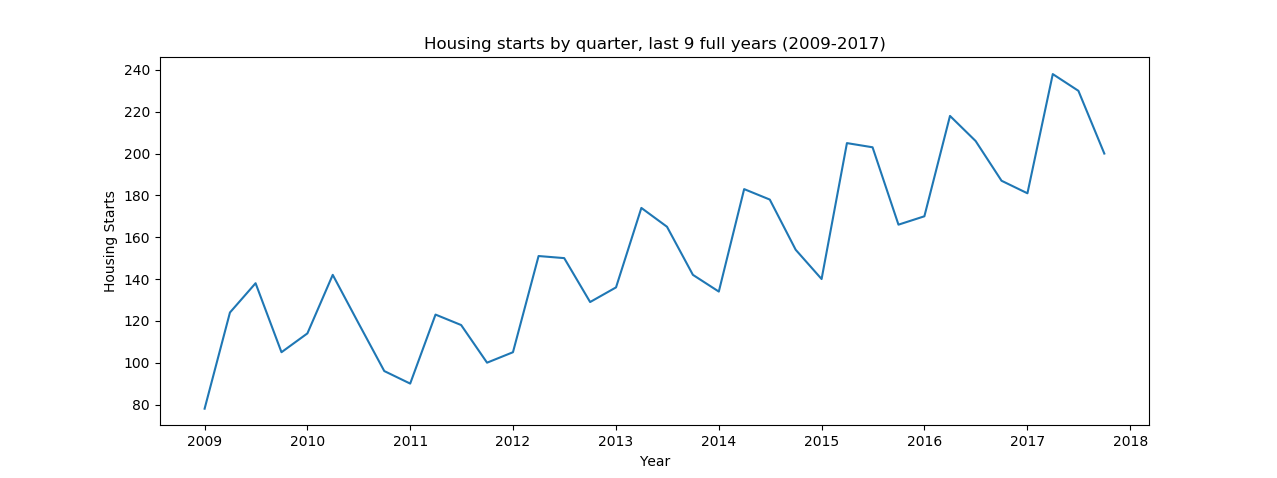Let's find a least-squares regression line to forecast demand, and add the calculated predictions to the dataframe, then plot it:

```# This creates a period column for the last nine full years
last_9_years_df = df[-39:-3]
last_9_years_df = last_9_years_df.reset_index()
last_9_years_df['Period'] = last_9_years_df.index + 1

# This calculates the OLS regression for the last nine full years
import statsmodels.api as sm
y = last_9_years_df['HOUST1FQ']
results = sm.OLS(y,X).fit()

# This creates a column in the dataframe using the results of the OLS regression
c, m = results.params
last_9_years_df['prediction'] = c+m*last_9_years_df['Period']

# This creates a line plot for the last nine full years PLUS the regression prediction
plt.plot(last_9_years_df['observation_date'],last_9_years_df['HOUST1FQ'], label='Actual Demand')
plt.plot(last_9_years_df['observation_date'],last_9_years_df['prediction'], color='red', label='OLS Regression')
plt.xlabel('Year')
plt.ylabel('Housing Starts')
plt.title('Housing starts by quarter, last 9 full years (2009-2017)')
plt.legend()
plt.show()
```

Which produces: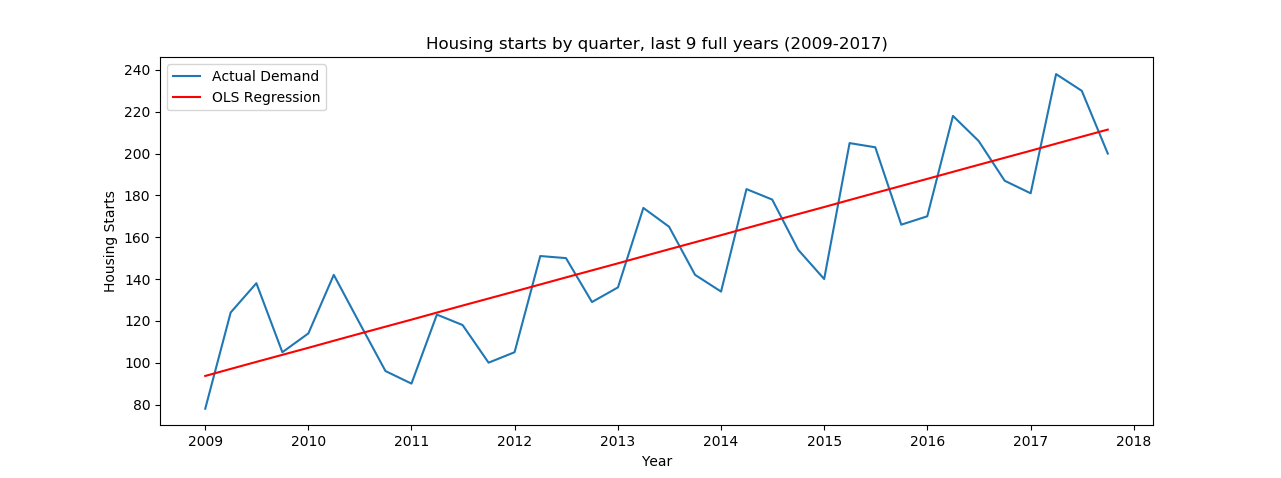Now we have to calculate a seasonal factor. We do this by looking at demand for each period and compare it to the forecast for each period, and average over all cycles. Since we've already added a column for season in the dataframe, we can just sum the actual demand grouped by season and divide it by the predicted demand grouped by season:

```# This calculates the seasonal factor for each season, adjusts the prediction by that amount, and tacks that on as a new column in the dataframe
actual_demand = last_9_years_df['HOUST1FQ'].groupby(last_9_years_df['season']).transform('sum')
predicted_demand = last_9_years_df['prediction'].groupby(last_9_years_df['season']).transform('sum')

# This plots the last nine years demand, regression prediction, as well as the adjusted prediction
plt.plot(last_9_years_df['observation_date'],last_9_years_df['HOUST1FQ'], label='Actual Demand')
plt.plot(last_9_years_df['observation_date'],last_9_years_df['prediction'], color='red', label='OLS Regression')
plt.xlabel('Year')
plt.ylabel('Housing Starts')
plt.title('Housing starts by quarter, last 9 full years (2009-2017)')
plt.legend()
plt.show()
```

Which produces: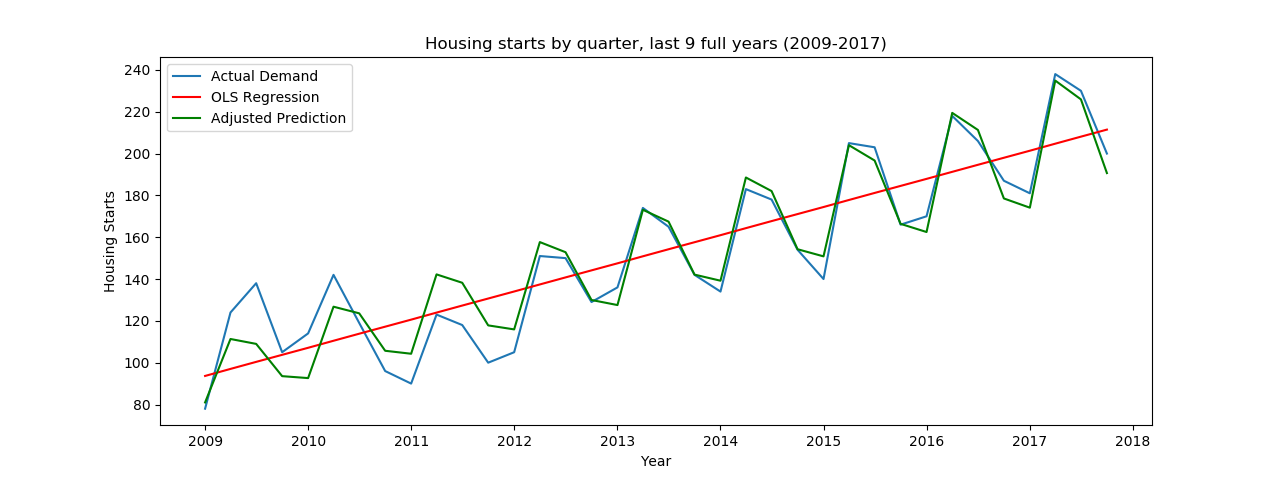We can check the improvement in forecast by checking the mean squared error. I'll use scikit-learn's formula to make it easy to calculate:

```# This calculates and compares the mean squared error for each forecast method
from sklearn.metrics import mean_squared_error
mse_linear_regression = mean_squared_error(last_9_years_df['HOUST1FQ'], last_9_years_df['prediction'])